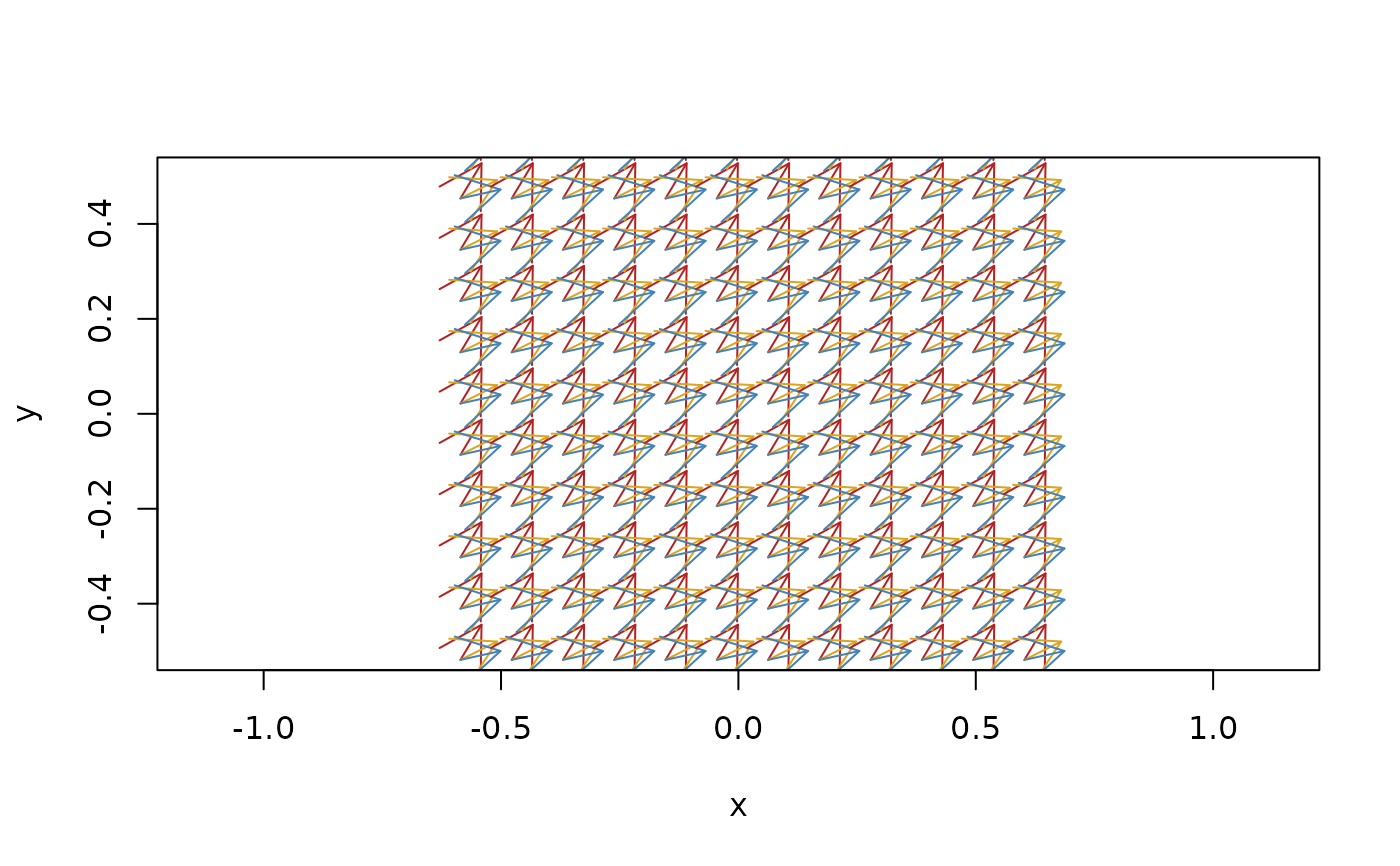Directions are vectors where you have forgot about the length. They are used much in the same way as normalised vectors (vectors with a magnitude of 1), but since vectors cannot be normalized while maintaining exactness it is preferable to simply have a data type where you ignore the magnitude. The direction can be flipped by taking the negative. 2 dimensional directions can be considered as angles and can thus be sorted and compared. The same is not true for directions in 3 dimensions.

direction(..., default_dim = 2)

is_direction(x)

between(x, d1, d2)

as_direction(x)

## Arguments

...

Various input. See the Constructor section.

default_dim

The dimensionality when constructing an empty vector

x

A direction vector or an object to convert to it

d1, d2

direction vectors to relate to

## Value

a euclid_direction vector

## Constructors

2 and 3 dimensional directions

• Providing 2 or 3 numerics will create directions with the given delta values. (2 numerics will give 2 dimensional directions, 3 will give 3 dimensional directions).

• Providing vectors will construct directions as the direction of the given vectors.

• Providing lines will construct directions as the direction of the given lines.

• Providing rays will construct directions as the direction of the given rays.

• Providing segments will construct directions as the direction of the given segments.

Other Geometries: circle(), iso_cube(), iso_rect(), line(), plane(), point(), ray(), segment(), sphere(), tetrahedron(), triangle(), vec(), weighted_point()

Other Arrows: vec()

## Examples

# Constructions
d <- direction(sample(10, 3), sample(10, 3))
d
#> <2D directions >
#>  <dx:2, dy:9> <dx:7, dy:2> <dx:1, dy:8>
plot(d, col = c("firebrick", "goldenrod", "steelblue"))# flipping the direction
-d
#> <2D directions >
#>  <dx:-2, dy:-9> <dx:-7, dy:-2> <dx:-1, dy:-8>

# Relations
d < d
#>  FALSE

min(d)
#> <2D directions >
#>  <dx:7, dy:2>

sort(d)
#> <2D directions >
#>  <dx:7, dy:2> <dx:2, dy:9> <dx:1, dy:8>

between(d, d, d)
#>  TRUE